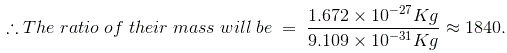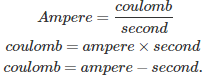# MCQs on Electric Circuits

##### Page 16 of 63. Go to page 1 2 3 4 5 6 7 8 9 10 11 12 13 14 15 16 17 18 19 20 21 22 23 24 25 26 27 28 29 30 31 32 33 34 35 36 37 38 39 40 41 42 43 44 45 46 47 48 49 50 51 52 53 54 55 56 57 58 59 60 61 62 63
01․ The fixed circular paths around the nucleus are called
orbits.
orbitals.
mesons.
nucleus.

We can call the circular path around the sun as an orbit. Like that only, nucleus is surrounded by electrons as circularly. That is why we are calling that fixed circular path as an orbit, too.

02․ Atoms with fewer than 4 valence electrons are
insulator.
semi-conductor.
conductor.
none of these.

Atoms with less than 4 valence electrons will lose electrons to revert back to the previous full energy level of electrons. This happens in conductors only. It can lose valence electrons easily and conduct current.

03․ Atoms have no electric charge because they
have an equal number of charged and non charged particles.
have neutrons in their nuclei.
have an equal number of neutrons and protons.
have an equal number of electrons and protons.

Because, equal number of negative electrons and positive protons would give a result as neutral. That is why an atom does not have electric charge.

04․ What is the mass number of an element that has 19 protons, 19 electrons, and 20 neutrons?
19.
39.
38.
20.

The mass number of an element is equal to the addition of the number of protons and neutrons. ( ∴ p+n=19+20 = 39 ).

05․ The electron is lighter than a proton by a factor of about
18.4.
184.
1840.
18400.

The mass of electron is 9.109 × 10 - 31 Kg, whereas the mass of a proton is 1.672 × 10 - 27 Kg.06․ The charge of an electron is
- 1.602 × 10 - 19 Coulombs.
- 1.675 × 10 - 27 Coulombs.
- 1.672 × 10 - 27 Coulombs.
- 9.109 × 10 - 31 Coulombs.

The charge of an electron is - 1.602 × 10 - 19 Coulombs.

07․ The mass of a proton is
1.675 × 10 - 27 Kg.
1.672 × 10 - 27 Kg.
1.602 × 10 - 19 Kg.
1.609 × 10 - 31 Kg.

A proton carries a positive charge and its mass is 1.672 × 10 - 27 Kg.

08․ The mass of a neutron of atom is
1.675 × 10 - 27 Kg.
1.672 × 10 - 26 Kg.
1.602 × 10 - 19 Kg.
1.609 × 10 - 31 Kg.

A neutron carries no charge and its mass is 1.675 × 10 - 27 Kg.

09․ Which of the following is the heaviest?
Molecule.
Atom.
Electron.
Proton.

Molecule contains atom, atom contains electrons and protons.

10․ Ampere second is the unit of
conductance.
power.
energy.
charge.

Current is transfer electrical charge per second.<<<1415161718>>>